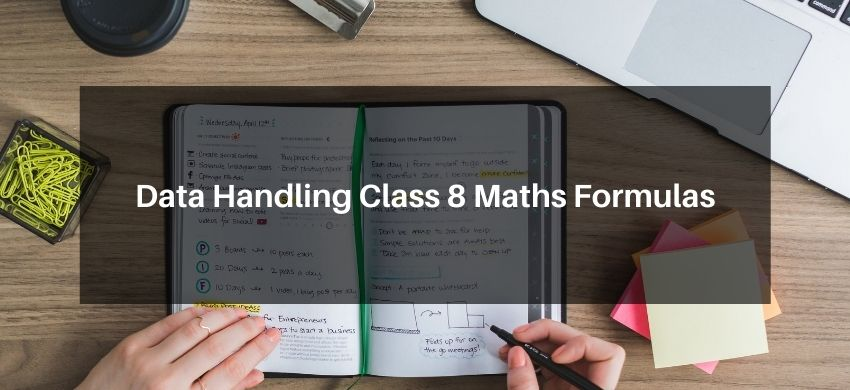Download the eSaral app and start learning from Kota's top IITians and doctors.

# Data Handling Class 8 Maths FormulasHey, students are you looking for Data Handling Class 8 Maths Formulas? If yes. Then you are at the right place. In this post, I have listed all the formulas of Data Handling class 8 that you can use to learn and understand the concepts easily.

If you want to improve your class 8 Math, Data Handling concepts, then it is super important for you to learn and understand all the formulas.

By using these formulas you will learn about Data Handling.

With the help of these formulas, you can revise the entire chapter easily.

## Data Handling Class 8 Maths Formulas

• Data: Numerical observations collected by an observer is called data (raw data).
• Frequency: The number of times an observation occurs in the given data is called the frequency of the observation.
• Frequency Distribution: A way of presenting data that exhibit the values of the variable and corresponding frequencies is called a frequency distribution.
• Range: The difference between the highest and the lowest values of observation is called the range. i.e.,

Range = Highest observation – Lowest observation
• Class-mark: The mid-point of a class-interval is called class-mark.

Class-mark $=\frac{\text { Upper limit + Lower limit }}{2}$
• Histogram: Grouped data can be presented using the histogram. A histogram is a type of bar diagram, where the class intervals are shown on the horizontal axis and the height of the bars show the frequency of the class interval. Also, there is no gap between the bars as there is no gap between the class intervals.
• Pie-chart or Pie-diagram: A pie-chart is a pictorial representation of the numerical data by non¬intersecting adjacent sectors of the circle such that area of each sector is proportional to the magnitude of the data represented by the sector.

Central angle $=\frac{\text { Value of a particular mode }}{\text { Sum of the value }} \times 360^{\circ}$
• Probability: Probability is the possibility and impossibility of an event (E).

Probability of an event $(E)=\frac{\text { Favourable outcomes }}{\text { Total number of possible outcomes }}$ and $P(E)+P($ not $E)=1$, i.e., $0 \leq P(E) \leq 1$

Probability of an event can have a value from 0 to $1 .$

The probability to a sure event is 1.

Probability of an impossible event is 0.
• Random Experiment: A random experiment is one whose outcome cannot be predicted exactly in advance.
• The mid-value of a class is called the class mark.

Class Mark $=\frac{\text { Lower limit + Upper limit }}{2}$
• Probability of an event $=\frac{\text { Number of outcomes that make an event }}{\text { Total number of outcomes of the experiment }}$

If you have any Confusion related to Data Handling Class 8 Maths Formulas, then feel free to ask in the comments section down below.

To watch Free Learning Videos on Class 8 Maths by Kota’s top Faculties Install the eSaral App